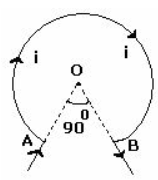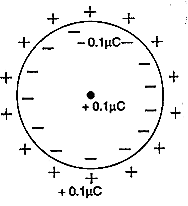# CBSE Class 12 Physics Chapter 4 Important Questions## myCBSEguide App

Complete Guide for CBSE Students

NCERT Solutions, NCERT Exemplars, Revison Notes, Free Videos, CBSE Papers, MCQ Tests & more.

CBSE Class 12 Physics Chapter 4 Important Questions. We know Physics is tough subject within the consortium of science subjects physics is an important subject. But if you want to make career in these fields like IT Consultant, Lab Technician, Laser Engineer, Optical Engineer etc. You need to have strong fundamentals in physics to crack the exam. myCBSEguide has just released Chapter Wise Question Answers for class 12 Physics. There chapter wise Extra Questions with complete solutions are available for download in myCBSEguide website and mobile app. These Questions with solution are prepared by our team of expert teachers who are teaching grade in CBSE schools for years. There are around 4-5 set of solved Physics Extra Questions from each and every chapter. The students will not miss any concept in these Chapter wise question that are specially designed to tackle Board Exam. We have taken care of every single concept given in CBSE Class 12 Physics syllabus and questions are framed as per the latest marking scheme and blue print issued by CBSE for class 12.

Class 12 Physics Extra Questions

## Moving Charges and Magnetism Class 12 Physics Practice Test

Class – 12 Physics (Moving Charges and Magnetism)

1. The magnetic field at the centre of the circular coil of radius 1 cm and carrying current of 4 A is1. {tex}\frac{8\pi}{3}{/tex} {tex} \times {\rm{1}}{0^{ – {\rm{4}}}}{\rm{T}}{/tex}
2. {tex}\frac{8\pi}{3}{/tex} {tex} \times {\rm{1}}{0^{ – {\rm{5}}}}{\rm{T}}{/tex}
3. {tex}{\rm{2}}\pi {\rm{ }} \times {\rm{1}}{0^{ – {\rm{5}}}}{\rm{T}}{/tex}
4. {tex}{\rm{2}}\pi {\rm{ }} \times {\rm{1}}{0^{ – {\rm{4}}}}{\rm{T}}{/tex}
2. Magnetic moment of a single-turn current loop of aluminium wire of area A,carrying a current I in a wire of diameter d is

2. μIA
3. IA/d
4. IA
3. According to Ampere’s circuital law

1. ​​​​​​{tex} \oint \vec{B} . \vec{dl} = I{/tex}
2. {tex} \oint \vec{B} . \vec{dl} = 2\pi\mu_0 I{/tex}
3. {tex} \oint \vec{B} . \vec{dl} = \pi\mu_0 I{/tex}
4. {tex} \oint \vec{B}. \vec{dl} = \mu_0 I{/tex}
4. The scale of a galvanometer of resistance {tex}100\Omega{/tex} contains 25 divisions. It gives a deflection of one division on passing a current of {tex}4 \times {10^{ – 4}}A{/tex}. The resistance in ohms to be added to it so that it may become a voltmeter of range 2.5 volt is:

1. 100
2. 250
3. 300
4. 150
5. A wire of length L carrying current i is placed perpendicular to the magnetic induction B. The total force on the wire is
1. LB/i
2. iL/B
3. iLB
4. iB/L
6. What are the units of magnetic permeability?

7. Under what conditions is the force acting on a charge moving through a uniform magnetic field minimum?

8. A long straight wire carries a current of 35 A. What is the magnitude of the field B at a point 20 cm from the wire?

9. Write SI unit of magnetic field {tex}\overrightarrow B {/tex}.

10. Two long parallel straight wires X and Y separated by a distance of 5 cm in air carry currents of 10 A and 5 A respectively in opposite directions. Calculate the magnitude and direction of the force on a 20 cm length of the wire Y.

11. An electron is moving along +ve x-axis in the presence of uniform magnetic field along +ve y-axis. What is the direction of force acting on it?

12. Two parallel coaxial circular coils of equal radius ‘R’ and equal number of turns ‘N’, carry equal currents ‘I’ in the same direction and are separated by a distance ‘2R’. Find the magnitude and direction of the net magnetic field produced at the midpoint of the line joining their centres.

13. An electron traveling west to east enters a chamber having a uniform electrostatic field in north to south direction. Specify the direction in which a uniform magnetic field should be set up to prevent the electron from deflecting from its straight line path.

14. Define current sensitivity and voltage sensitivity of a galvanometer. Increasing the current sensitivity may not necessarily increase the voltage sensitivity of galvanometer. Justify

15. If a piece of metal has a charge {tex} + 0.1\mu C{/tex} and is placed inside a hollow metal sphere of radius 20 cm (without touching it), what is the potential of the sphere? What will the potential of the sphere become, if

1. the sphere is temporarily earthed and then left insulated,
2. the metal subsequently touched the inside of the sphere?

Class – 12 Physics (Moving Charges and Magnetism)

1. {tex} {\rm{2}}\pi {\rm{ }} \times {\rm{1}}{0^{ – {\rm{5}}}}{\rm{T}}{/tex}
Explanation: i=4 A, r= 10-2 m
Magnetic field = {tex}\frac{\mu_0i}{2r}\times\frac\theta{360^0}{/tex}
{tex}=\frac{4\mathrm\pi\times10^{-7}}2\times\frac4{10^{-2}}\times\frac{90}{360}\;=2\mathrm\pi\times10^{-5}\;\mathrm T{/tex}
1. IA
Explanation: The torque experienced by a current carrying coil of N turns placed in a magnetic field {tex}\vec \tau = NI\vec A \times \vec B{/tex}.
For N =1, {tex}\vec \tau = I\vec A \times \vec B{/tex} Comparing with torque experienced by a electric dipole
we get {tex}\overrightarrow{M}\times\overrightarrow B=I\overrightarrow{A}\times\overrightarrow B{/tex} hence {tex}\overrightarrow{M}=I\overrightarrow{A}{/tex}.
1. {tex} \oint \vec{B}. \vec{dl} = \mu_0 I{/tex}
Explanation: The expression for Ampere’s circuital law is {tex}\oint {\vec B \cdot d\vec l = } {\mu _0}I{/tex}
Where I is the current included in the surface bounded by the line integral.
1. 150
Explanation: {tex}R=\frac V{I_g}-G{/tex}
V = 2.5 V ; G = 100 Ohm ; {tex}I_g\;=0.01\;A{/tex}
solving we get
R = 150 Ohm
1. iLB
Explanation: Magnitude of the Force experienced by a current carrying conductor placed in a magnetic field is {tex}ILB\sin\theta{/tex} . If the angle between the directions of the current and the magnetic field is 90⁰, F= iLB
1. Tesla metre / ampere (TmA-1).
2. When a charge moves parallel or antiparallel to the direction of the magnetic field, the force acting on it is zero or minimum.
3. Given, I = 35 A, r = 20 cm, = 0.2 m
{tex}B = \frac{{{\mu _0}I}}{{2\pi r}} = \frac{{4\pi \times {{10}^{ – 7}} \times 35}}{{2\pi \times 0.20}}T{/tex}
{tex} = 3.5 \times {10^{ – 5}}T{/tex}
4. SI unit of magnetic field is tesla (T)
5. By using formula,
{tex}F = \frac{{{\mu _0}{I_1}{I_2}l}}{{2\pi r}}{/tex}
{tex} = \frac{{4\pi \times {{10}^{ – 7}} \times 10 \times 5 \times 0.20}}{{2\pi \times 0.05}} = 4 \times {10^{ – 5}}N{/tex}
The direction of force is perpendicular to the length of wire Y and acts away from X.
6. The direction of the force is along -ve z-axis.
7. Magnetic field at the midpoint due to loop 1.
{tex}{B_1} = \frac{{{\mu _0}i{R^2}}}{{2{{\left( {{R^2} + {R^2}} \right)}^{\frac{3}{2}}}}}{/tex} acting towards right
Magnetic field at the mid point due to loop 2.
{tex}{B_2} = \frac{{{\mu _0}i{R^2}}}{{2{{\left( {{R^2} + {R^2}} \right)}^{\frac{3}{2}}}}}{/tex} acting towards right
Total magnetic field at the mid point
B = B1 + B2
or {tex}B = \frac{{{\mu _0}i{R^2}}}{{{{\left( {2{R^2}} \right)}^{\frac{3}{2}}}}}{/tex}
which is acting towards right.
8. The electrostatic field is directed towards south. Since the electron is a negatively charged particle, therefore, the electrostatic field shall exert a force directed towards north. So, if the electron is to be prevented from deflection from straight path, by the magnetic force on the electron should be directed towards south. Now {tex}{\overrightarrow F _m} = – e(\overrightarrow v \times \overrightarrow B ) \cdot {\overrightarrow F _m}{/tex} is towards south, {tex}\overrightarrow v {/tex} is due east. Applying Fleming’s left hand rule we find that magnetic field {tex}\overrightarrow B {/tex} should be in the vertically downward direction.
9. The definition of current sensitivity and voltage sensitivity are given
{tex}\rightarrow{/tex} Let the deflection produced in applying voltage V is {tex}\alpha{/tex} then
Voltage sensitivity {tex} = \frac{\alpha }{v} = \frac{{NBA}}{{kR}}{/tex}
The voltage sensitivity may be increased by (i) increasing, N, B, A (ii) decreasing k and
Current sensitivity {tex} = \frac{{NBA}}{k}{/tex} can be increased by
(i) increasing NBA (ii) decreasing k.
Hence increasing the current sensitivity may not necessarily increase the voltage sensitivity of a galvanometer.
10. On the inner and outer sphere’s surface charges of {tex} – 0.1\mu C{/tex} and {tex} + 0.1\mu C{/tex} are induced respectively. The potential of the sphere relative to earth is determined solely by the outer surface charge q.
{tex}\therefore V = \frac{1}{{4\pi {\varepsilon _0}}}\frac{q}{r}{/tex}
where r is the radius of the sphere
Now, {tex}V = 9 \times {10^9}N{m^2}{C^{ – 2}}\frac{{0.1 \times {{10}^{ – 6}}C}}{{0.2m}}{/tex}
= 4500 V

1. The potential of the sphere is momentarily reduced to zero when it is earthed. The positive charge on the outer surface disappears but the induced negative charge inside remains. Therefore, the potential of the sphere is zero.2. The induced negative charge is neutralized when the metal touches the sphere and no charge remains on the metal or sphere. Both are at the same potential i.e. zero potential.

## Chapter Wise Extra Questions of Class 12 Physics Part I & Part II## Test Generator

Create Papers with your Name & Logo